# Exciting Maths Addition Sums For KidsAddition sums are one of the simplest and interesting topics of Maths for kids in primary classes. Maths is an interesting subject and it is during the initial years that children learn mathematical concepts like Maths addition sums for the first time. Being one of the four basic operations of Arithmetic, addition is the process of adding one or more numbers to obtain a new total. Kids learn basic addition questions in the primary classes.

Addition forms an important part of Maths syllabus that is essential for kids learning process. In the primary classes, kids are taught simple addition sums like one-digit facts, Maths addition sums, double-digit Maths addition questions with numbers from 0-10, 0-20, 0-30, etc. Addition is referred through the following symbol (+) in mathematical terms.

Questions on Addition are very interesting to solve. Here are a few simple addition sums, questions and answers that young kids can find useful while studying Mathematics:

1. Single-digit Addition (Sums up to 10):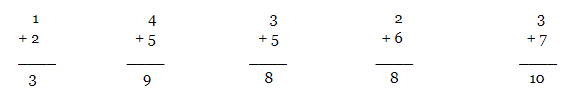1. Single-digit Addition (Sums up t0 20):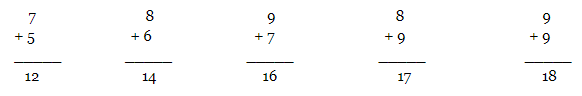1. Addition of two double-digit numbers (Sums up t0 30):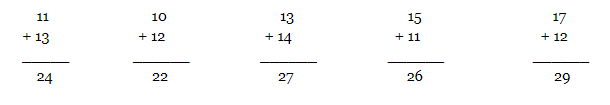1. Double-digit addition (Sums up to 100):

Steps to solve the addition of two double-digit numbers.

Example: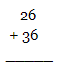Step 1: Add the ones place digits i.e. 6+6 = 12. This number is larger than 10. So write “2” and carry the “1”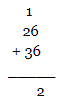Step 2: Add the tens place digits i.e. 1(carried)+2+3 = 6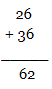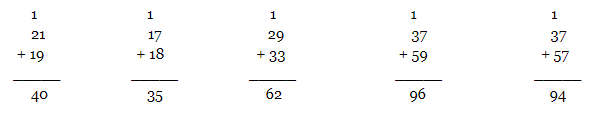1. Double-digit addition (Sums more than 100):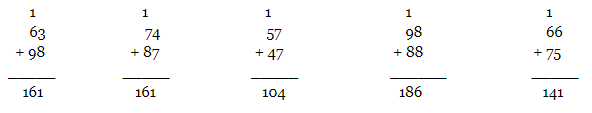1. One triple-digit and one double-digit addition: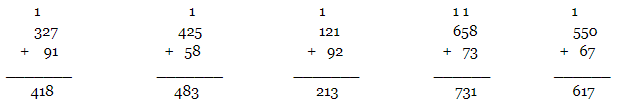1. Addition of two triple-digit numbers:

Steps to solve the addition of two triple-digit numbers.

Example: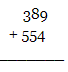Step 1: Add the ones place digits i.e. 9+4 = 13. This number is larger than 10. So write “3” and carry the “1”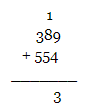Step 2: Add the tens place digits i.e. 1(carried)+8+5 = 14. This number is larger than 10. So write “4” and carry the “1”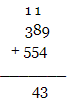Step 3: Add the hundreds place digits i.e. 1(carried)+3+5 = 9.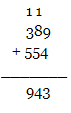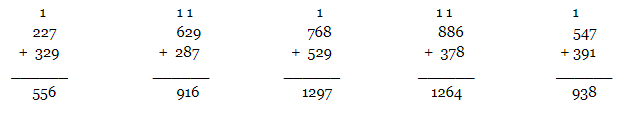### Resources for Kids

Listed below are the class-wise Maths pages for your child:

It is between the ages 4 to 8 that kids learn to solve simple addition sums like adding single or double-digit numbers, column Maths addition questions, addition and subtraction questions, etc. We bring you a huge collection of useful resources. Keep an eye on BYJU’S website to explore more.

Learning Maths at such a young age is like a stepping stone for kids as it prepares them for higher classes. You can find fun trivia questions for kids, poems, stories, essays, amazing GK Questions, NCERT Solutions, etc. on our website. Discover the best resources for your little one and give him/her the ultimate joy of learning.